### St Anne’s Academic Review 9 – 2019

The Design of Everyday Numbers
Matthew Lau – DPhil Candidate, Mathematical Institute
STAAR 9 – 2019, pp. 46-59

——————————–
Published: 13 October 2019
Review process: Open Peer Review

Abstract

What are numbers? Why are they everywhere? Why do they work so well? Can they be made better? Here, we will see where numbers come from and how to approach these questions.

Introduction

I am sure we would all agree that the wheel is a crucial invention that has pushed humanity forward. Hence, it is important to make sure that it is well designed. Wheels come in many different forms – from simple blocks of wood in a toy train to sophisticated multifeatured ones in a racing car – depending on various factors such as need, aesthetic, technology and cost. However, they all have the same underlying structure of a circular disc. Could this seemingly perfect circular disc design be improved? How should one go about customising wheels for a particular object? To answer these questions adequately and possibly reinvent the wheel, one would need to understand the underlying principles of wheels and their design.

Similarly, numbers are very influential. They seem to work smoothly, though we should question whether they are the right tools to have. Likewise, various systems of numbers have been developed. We have a few standard systems – from the natural numbers children first learn to count with, to the complex numbers used by physicists and engineers. There are also many lesser known systems, such as the octonions and the ordinal numbers. Moreover, each of these number systems has countless customisations that suit different purposes. In this article, we will try to understand where numbers come from, with a focus on the most ubiquitous systems: the natural numbers (with numbers like 0, 1, and 2) and the real numbers (with numbers like 1, -0.5 and π).

Comparing Apples and Oranges

We need some sense of what “numbers” mean in order to analyse them. We can begin with a glance at the concept of counting, which will shed light on this question and allow us to proceed with our investigation.

The phrase “comparing apples and oranges” expresses the idea that objects need to be sufficiently similar for meaningful comparisons. Contrary to this popular idiom, although the two fruits differ in many aspects, they certainly share the property of being countable. For example:

– “Three apples are more than two apples” is analogous to “Three oranges are more than two oranges.”

– “Putting three apples and two apples together, we have five apples” is analogous to “Putting three oranges and two oranges together, we have five oranges.”

Counting apples is equivalent to counting oranges: a person who can count apples would also be able to count oranges, and vice versa. In fact, counting has little to do with apples or oranges. Many types of objects can be counted: apples, oranges, fingers, days, dragons, etc. are all counted in an analogous way. By drawing this analogy and extracting the common underlying structure, we arrive at a system of “pure” counting with entities like “one”, “two”, “plus”, “greater than”, and so on (Figure 1). We can then make sense of sentences like “Three plus two is greater than four”, and reapply this knowledge to apples and oranges.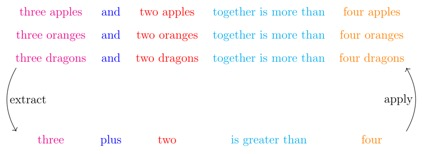Figure 1: An Analogy of Counting

In general, a number system extracts from certain concepts, which can then be studied abstractly and applied wherever these concepts are found. In other words, a number system is designed for certain concepts, just as a wheel is designed for certain transportations. A good wheel designer is one who is proficient in matching wheel designs with demands. On one hand, the designer, upon a particular request, can pinpoint and elaborate on the desired purposes and features for the wheel. On the other hand, the designer can construct wheel prototypes and analyse them for their properties. These are the two main skills that a wheel designer needs – given a demand, the designer would know the properties to seek for and come up with designs that has those properties. Similarly, in designing number systems, there are two main direction of approaches: concept-oriented and system-oriented.

In a concept-oriented approach, we aim to design number systems for a given concept. We are interested in the features and properties to seek for.

i) In what ways can the concept be formalised? A concept can have many facets and there can be multiple formalisations. As an example, time has various facets that result in different formalisations, such as:

– the notions of past, present and future.

– moments in time (e.g. the beginning of the universe).

– moments in a day (e.g. 4 PM).

– intervals of time (e.g. the year 2019).

ii) What assumptions are being made in a particular formalisation? Assumptions might not completely reflect our perceptions of the concept, but idealisations are often necessary for useful and insightful formalisations. Awareness of assumptions is critical for recognising potential flaws. For example, we can think of time going indefinitely far into the past, but this might be an issue when one is talking about the beginning of the universe.

iii) What formal features are available to the numbers? For example, the natural numbers have features such as comparison, addition and multiplication. Without any features, these numbers are just names (e.g. ‘Matthew’) that we cannot do very much with and the system would not be very useful.

– What notion in our concept would the given feature extract from? A feature would be more relevant if it had a corresponding notion. For example, addition corresponds to putting two collections together. However, an “unnatural” feature (e.g. adding 4 PM with 11 AM) might also be powerful and provide new insights about our concept.

– Can the given feature be amended and in what ways? A certain feature might be desirable, though it might conflict with other features or there could be multiple ways of amendment.

– What are the utilities in amending or not amending the given feature? Amending a feature can be useful, but can also create complications. For example, addition is often useful, but unnecessary for a tally counter which only lists the numbers in order.

iv) Can we develop a widely applicable standard system? Given the many choices of features, various systems are possible, which can cause confusion for potential users. Hence, a small number of standardised systems would be desirable. We have a few guidelines for choosing these:

– It is better to have a tool that is left aside than to not have the tool when it is needed. A standardised system should be inclusive enough to meet a wide range of needs. However, we should also be choosy: conflicts can occur, and simplicity is beneficial.

– Something often used or requested should be included, if possible.

– The system should be flexible. Ideally, users can obtain common alternative systems through easy adjustments to the standardised ones.

This is where system-oriented approach comes in to help us analyse and compare various number systems.

In a system-oriented approach, we investigate a number system by inspecting its extractions, applications, and mathematical properties. This system would then be available for building and understanding prototypes when we try to design number systems for a concept.

i) What are some primary concepts that the system is extracted from? In general, a number system can be extracted from many concepts and it would be difficult to list them all. However, a system is usually associated with a few prototypical ones. For example, the natural numbers are associated with counting.

ii) What modifications to the system are available? How does the system compare to other systems? A number system can be tailored for specific usage or similar concepts (e.g. the natural numbers can be modified to count days of the week, which are limited to seven). This also indicates the flexibility, capabilities and limitations of the system.

iii) How are the entities in the system represented? Representations are necessary for computation, recording and communication. Effective representations are important for efficient and intuitive usage.

iv) What is the collection of axioms (i.e. rules) that characterises the system? Having a collection of axioms is essential, since:

– To know whether the system can be extracted from a concept, we only need to check the concept against a collection of axioms.

– Axioms allow us to describe the system directly, which is particularly important for teaching and implementations in computers.

– It is convenient to create modifications and extensions by dropping, adding and changing axioms.

– Axioms prevent mistakes since they tell us for what we are allowed to do when using the system.

– From the axiom, we can derive handy tricks and facts for more efficient usage.

By combining the concept-oriented and system-oriented approaches, we should be able find number systems that extract from a given concept. Let us come back to our guiding question: where do the natural numbers and the real numbers come from? As we will see in the next few sections, they are designed for the concepts of counting and proportions, respectively.

Systems for Counting

We shall now have a more detailed look at counting within the framework of a concept-oriented analysis. We have a type of object (e.g. apples) which has a smallest unit (e.g. an apple) that we would like to count. Various formalizations are possible. Here, we will focus on the formalization where a number in our system would represent the “quantity” of a collection of such objects (e.g. “Three” represents “a collection of three apples”.) There are many assumptions to be made.

We will list a few important and perhaps subtle ones.

i. We suppose that it is clear what the smallest unit of counting is. It is perhaps possible to use our system to count apples that are sliced, burnt, rotten, blended, etc., though we leave it for the user to decide on an appropriate unit of counting in each of these contexts.

ii. We assume that, for example, we can have a collection of just one apple. This might not be the case if, for example, we are counting trees in forests, which would necessarily have more than an individual (since a forest has multiple trees).

iii. We expect that, for example, we can always add an apple to any given collection of apples to get another collection. This might not be the case if, for example, we assumed that the apples are to be put into a basket of a fixed size.

iv. We expect that, for example, we cannot add a few apples to a collection of apples and end up with the same number of apples. This might not be the case if, for example, we naively try to count blended apples: two apples blended together could be the same as just one big blended apple.

Having decided on the formalization and the assumptions, we will now look at a few features available to the numbers. For each feature, we will address the three questions as in the concept-oriented approach.

I. Succession.

– Each number can have a successor: the next number after it. Taking the successor corresponds to adding an object to a collection (Figure 2).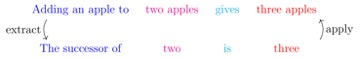Figure 2: Succession corresponds to adding an object.

– There is only one way to include the feature of succession – the assumptions specified above give restrictions as to how succession should behave. For example, we expect to get a different size by adding an object to a collection, so we might have an axiom that says that the successor of a number should not be itself (Figure 3).Figure 3: Rules for succession come from our assumptions.

– Succession is an essential feature. Many other features are built on top of it. It is useful and easy to understand – it is the feature that children first learn about.

– It is quite clear what addition corresponds to: the size given from putting two collections together.

– Addition can be defined by using successors. In fact, it is an extension of succession, which is essentially “adding by one”.

– Addition is fundamental to the applicability of the number system. It allows us to determine the size of a collection by partitioning it into multiple sub-collections and summing their sizes. On the other hand, there is utility in a system with succession but without addition, such as the use of a tally counter for tracking arrivals.

III. Multiplication.

– We certainly use multiplication to count in practice. However, it is not always clear what multiplication corresponds to – what should two apples “times” three apples mean? To have multiplication, we actually need a more involved extraction process, which we will look at later in this article.

– Multiplication can be defined with successors.

– Similar to addition, multiplication is fundamental, though it can be complicated.

IV. Zero.

– There is little ambiguity as to what zero corresponds to: an empty collection.

– Zero behaves well with standard features (e.g. succession, addition, multiplication, etc.).

– Having zero is often useful or even necessary. For example, zero (or something synonymous) would appear in the record for a student who never attended any lectures. On the other hand, it can also be useful to teach without zero and there are many instances where zero is irrelevant, such as when we are not interested in keeping track of empty collections.

V. Negative numbers.

– It is fairly clear what negative numbers correspond to: negative two represents “missing two objects”.

– As with zero, negative numbers can be included with no issues.

– The motivation for negative numbers is similar to that for zero, though the utility for not having negative numbers is more apparent. For example, it would be quite tricky for an attendance record to have a negative number for a student.

VI. Infiniteness.

– The size of an “unlimited” collection would be infinite.

– We will not go into details regarding the notion of infinity. What should be noted is that infinity can be incorporated in multiple ways.

– On one hand, features related to infinity are often avoided or not used and make the system more complicated. On the other hand, infinity is a very practical and indispensable notion, as used in disciplines such as physics and computer science.

Having glanced at several available features, we would like to develop a standardised number system for counting. Here, we should think from a system-oriented perspective to understand and compare various number systems. However, we will be skipping over the technical details and only make some important notes. One possible choice would be the succession system which only has the succession feature: the most ubiquitous feature across different contexts. The succession system is simple and contains the necessary functionality for building other common concepts. However, counting often involves much more than succession. The natural numbers with their usual operations would also be a good proposal – addition and multiplication have clear meanings for counting and constitute a more well-rounded toolkit. With a system-oriented analysis on the natural number, we would come to these conclusions:

i) The system of natural numbers is very flexible. Many other counting-related systems – trees in forests, apples in a basket, missing items, infinity – can all be built from the natural numbers.

ii) The natural numbers have effective representations. We will come back to this topic later.

iii) The assumptions we have made above regarding counting should guide us in our choice of axioms, though we will not go into details here.

iv) The natural numbers can be built from the succession system. This has two important implications:

– Extraction of the natural numbers can be broken down into two steps. Firstly, we need to ensure that the target concept (e.g. counting) does indeed instantiate succession as specified by the axioms for the succession system. Secondly, we need to make sure that other features, like addition and multiplication, built from succession, do in fact correspond to the notions that we anticipate.

– Wherever we find the succession system, we can apply the extra features from the natural numbers. This might lead to surprising, and possibly unintended, results. For example, we can “multiply” apples, though it is not clear what this means; this issue is addressed in the next section.

With these points in mind, we would reasonably suggest the natural numbers as the standardised system for counting. Indeed, we have been using them to count for centuries.

Remark: One might question whether “natural numbers” should refer to a system with or without zero. This is an important question to resolve, at the very least to avoid miscommunication. However, this is largely a matter of convention and the question is left to interested readers.

In this section, we have an outline of how the natural numbers become the standardised number system for counting. Many concepts involving counting in some manner, which is why we see the natural number applied everywhere. Next, we would like to see where the real numbers come from. However, we first need to address couple of issues.

One Number, Two Systems

Let us try to extract numbers from the positions in a race. We use “1”, “2”, “3”, etc. to respectively represent “first in the race”, “second in the race”, “third in the race”, etc. We then come to the succession system: the first is followed by the second, which is followed by the third, and so on.

Suppose I finish three places after the second place. What position in the race am I at? By using addition borrowed from the natural numbers, we have that 3+2=5. It is tempting to conclude that I came fifth in the race. The answer is correct, though the reasoning is flawed.

– In the expression “3+2”, we were trying to use “3” to represent “third after” though we actually meant for “3” to represent “third in the race”.

– How should we interpret “third in the race” plus “second in the race”? Knowing who are third and second in the race generally tell us little about the fifth place, which might not even exist!

The issue is that “3” is confusingly being used to represent multiple things simultaneously: “third in the race” and “third after”. In fact, we are working in a system with two subsystems: a succession system that represents positions in a race and an addition system that represents relative positions (Figure 4).Figure 4: Positions (Represented by the Succession System)
And Relative Positions (Represented by the Addition System)

Furthermore, we have two types of additions: one within the addition system (Figure 5) and another one between the two subsystems (Figure 6), where we reach from one position to another by adding a relative position.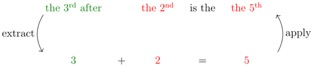Figure 6: Positions (Represented by the Succession System)

Similarly, when using multiplication to count collections of apples, we have a pair of subsystems: an addition system for counting apples in a collection and a system of natural numbers for counting collections (Figure 7 and 8).Figure 7: Apples (Represented by the Addition System)
Multiplied by Collections (Represented by the Natural Numbers)Figure 8: Multiplication of Collections in the Natural Numbers

There are two important notes to take away here. Firstly, an extraction can generally involve multiple number systems (and possibly multiple copies of the same system) with interactions among them. Secondly, the successor system, the addition system, and the natural numbers are related but distinct systems. For example, there is a number called “five” in each of these systems. We can draw analogies between these numbers that happen to share the same name, but they are ultimately different numbers as they express different meanings.

Systems for Proportions

We saw that the natural numbers form the standardised system for counting. Another well-known system is the real numbers, which is the standardised system for proportions. We should similarly take the concept-oriented approach on the concept of proportion and arrive at the real numbers. However, we will be glossing over most of the details as the procedure is parallel to that for counting but has much more complexity and technicality. We shall focus on the formalisation process and the desired features, which would be indicative of how the real numbers emerge from the notion of proportion.

Let us suppose that we have a train station with a straight train track that extends indefinitely to the west and to the east, imagining hypothetically that the Earth is flat. Firstly, we shall formalise the concept of position (Figure 9).

– “0” represents where the track meets the station.

– “1km E” represents the position on the track that is 1 kilometre east of the station.

– “4.276km E” represents the position on the track that is 4.276 kilometres east of the station.

– “2km W” represents the position on the track that is 2 kilometres west of the station.Figure 9: Positions on a Train Track

We would then extract a system of numbers permitting comparisons. For example, 2km W is to the east of 4km W. However, we do not have features like addition or multiplication. Indeed, it does not mean much to “add” positions: what position would the sum of Hong Kong and Oxford be? We can then formalise the concept of movements.

– “0” represents “staying still”.

– “3km E” represents “moving 3 kilometres eastward”.

– “0.889km W” represents “moving 0.889 kilometres westward”.

We now arrive at a system where we can compare and add (Figure 10), though we are unable to multiply.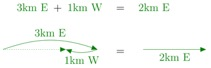We can also move from one position to another by “adding” a movement (Figure 11).Figure 11: Positions Added by Movements

Finally, we can formalise the concept of proportions.

– “4” represents “scaling a movement by a factor of 4”.

– “-0.5” represents “scaling a movement by a factor of 0.5 and switching direction”.

– “0” represents “scaling to no movement”.

As expected, we can scale a movement by “multiplying” with a proportion (Figure 12).Figure 12: Movements Scaled by Factors

We have a system representing proportions where we can add, subtract, multiply and divide (Figure 13).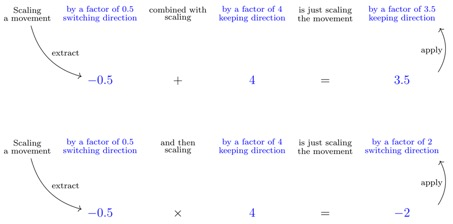Figure 13: Mathematical Operations on Scales

The concept of proportion comes up in many situations besides our train track example. From architecture to music, from sociology to physics, proportions are everywhere. With a variety of assumptions and decisions in the concept-oriented approach, we would reach the real numbers as the standardised system for proportions.

One query in making our assumptions does merit particular attention. Should we include infinitesimal proportions? In the case of a train track, to have infinitesimal proportions is to have small undetectable movements. From the perspective of measurements, there is no need for minuscule movements, as such things will never be recorded; this is the view held by the system of real numbers, which has no infinitesimals. However, they formalise the intuition of a very small object or movement and are useful for describing things from tiny superheroes to calculus.

Representation of Numbers

Good representations of numbers are crucial for their applicability. Here, we will focus on the representation of real numbers. Natural numbers are generally represented as a subsystem of the reals. Numbers have been represented in many ways in various civilisations and in various mediums (from carvings to electrical charges). However, aside from variations in syntax, the place–value notation has become the world standard.

i) The place–value notation requires a chosen natural number greater than one as the base. Ten is the commonly-chosen base, and we will use it here for illustration.

ii) There are ten digits (such as “0”, …, “9” in Arabic numerals) to represent the numbers zero through nine along with additional symbols (such as “-”, “.” And “,”) for indicating negativity and position.

iii) In the representation of a number, digits are put in a sequence. Each position, based on where it appears in the sequence, is given a distinct weight that is a power of ten. A negative sign indicates that the number is negative. (Figure 14)Figure 14: Place-Value Notation for Real Numbers

iv) The sequence of digits may be infinite and symbols are used to indicate that the sequence is endless or repeats indefinitely (Figure 15).Figure 15: Numbers with Endless Digits

We will consider and discuss some characteristics of this notation.

i) It allows for a concise and precise representation of a large range of numbers. For comparison, consider the representation of natural numbers “one-by-one” using fingers, apples, grains of sand, etc.:

– One would have trouble representing large numbers, such as 10000, with fingers or apples.

– A few grains of sand can represent a small number while a heap can represent a large number. However, moving and counting sand grains is tedious: it would be tricky to preform precise calculations and comparisons.

ii) Many important numbers, such as π, would require infinitely many irregular digits and so they could only be approximated in this notation. In most practical instances, approximations of these numbers are quite sufficient. The place–value notation can simply be extended (with notations like “π”) when exact computations are required.

iii) The scale of numbers is made transparent and it is easy to compare numbers that are close by. For example, consider these numbers and their representations (Figure 16).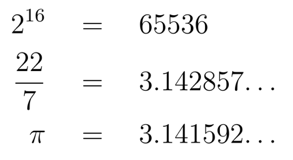Figure 16: Numbers in Various Representations

From the right-hand side, it is clear that the first number is at a much larger scale than the second, which is just a little bigger than the third. In contrast, this information is not laid out on the left.

i) It only requires a fixed number of specified symbols which is useful for memorisation and implementation in computers. In contrast, the words “ten”, “hundred”, “thousand”, etc. do not follow an easy pattern.

ii) Each digit is a symbol and is used the same way in all positions. In contrast, Roman numerals require multiple and distinct symbols to represent the same value at different positions. For example, “VI”, “LX“, “DC” represent “6”, “60”, “600” respectively.

iii) It allows for effective computations. The commonly-taught method of vertical calculation takes advantage of various mathematical tricks that are made applicable by this representation. Furthermore, these computational methods only require a fixed amount of memorisation (e.g. the multiplication table), which is again useful for computers. For example, we can see below how summing 67 with 324 can be done neatly with the standard method, saving a lot of tedious steps (Figure 17).Figure 17: An Addition Method Using Various Tricks

iv) Numbers in weaker subsystems (such as the succession system and the addition system) are represented in the exact same way. These differences are somewhat respected in languages (e.g. “third”, “three” and “thrice”), but they are not indicated in mathematical computations. Introducing and using notations (such as “1st”) for distinguishing numbers in different systems would potentially improve understanding and reduce errors in reasoning.

v) It requires a choice of a base. Various bases have different uses. There are a few factors to consider.

– How intuitive is the base? This is relevant for humans but not so much for computers.

– How large or small is the base? Smaller bases have less memorisation and easier computations, at the cost of longer representations. For example, the multiplication table in base-two only has three entries (0×0=0, 1×0=0 and 1×1=1) while the representation for a thousand is long (1111101000).

– How divisible is the base? When trying to share the bill for a meal evenly, three people often run into issues. This is essentially because the common monetary systems use base-ten, in which one third has an infinite number of digits (0.333…). In contrast, ten is a multiple of five, so base-ten is good for divisions by five. A base with many small factors would be desirable: divisions of natural numbers are less likely to require infinitely many digits and multiplication tables are easier to memorise.

Modern computers, which speak the language of on-and-off signals, are most suitable for a base-two notation. However, there might be a “chicken and egg” dilemma here: base-two computations are easy to implement, and this would conversely promote the use of on-and-off signals in computers.

Which base is best for humans? Mediums (such as text and speech) used by humans have room for more digits, allowing for a larger base. At the same time, we are not terribly good at large amounts of precise memorisation and a base too large might be difficult to use. Ten is probably a decent size and is quite intuitive since each of us typically has ten fingers. However, it has the drawback of not being divisible by three. There are many common situations in which one needs to divide by three, so bases like six and twelve might be better alternatives. Regardless, it would be a tremendous task to have societies around the world switch to a different base, and the benefits might be insignificant, as computers are gradually performing more computations for us. I shall leave the rest of this debate for the reader.

Representations of numerical operations are important as well. We will look at two factors in particular.

i) To express the sum of two and three, we put a plus sign in-between the represented numbers: “2+3”. This is intuitive as the plus sign connects the two numbers and indicates that we should combine them. Nonetheless, other conventions are available. For example, the Polish notation puts the plus sign in front of the numbers (e.g. + 2 3) and this can be more efficient for computers.

ii) Brackets are used to indicate the order of operations in an expression. To avoid the clustering of brackets, it is helpful to have operation precedences. For example, the common standard is that multiplications are done before additions unless bracketed otherwise (Figure 18).

The expression 2×3+5

would mean (2×3)+5

and not 2×(3+5).

– Figure 18: Operation Precedence

This is a good convention because we can expand any complicated expression containing multiplications and additions into one that does not need any brackets (Figure 19).

((a+b) × (c+d)) + e can be rewritten as
a×c + a×d + b×c + b×d + e which is bracket-free.
– Figure 19: Brackets Removed by Expansions

In contrast, this is not the case if we take the convention of doing additions before multiplication. For example, it would not be possible to remove the bracket in the expression (a×b)+c.

Overall, we see that there are a lot of factors that contribute to the design of representations for number systems. Different notations are suited to different purposes (e.g. approximate vs. exact) and users (e.g. humans vs. computers). The current standard of notation, though not without its flaws, works well and will undoubtedly continue to be used to represent the real and natural numbers in the foreseeable future.

Conclusion

An essential part of humanity is our ability to conceptualize the things we observe and to build a large variety of systems for understanding such concepts. These systems are complex and diverse, but they ultimately share a lot of common subsystems. For example, counting and proportions underpin a lot of concepts, and it is therefore not surprising that their standard systems – the natural numbers and the real numbers – are so ubiquitous. It is undeniably useful and important to have such common systems for building other systems. Hence, we inevitably give them the label “number” that they deserve.

Numbers are everywhere since they are the standardised systems extracted from various primitive concepts. With works spanning across civilisations, mathematicians have provided us with effective and efficient number systems. However, it would be fallacious to use these numbers blindly. Many subtle assumptions and decisions are made in an extraction of a concept and the resulting system often involve a range of interacting subsystems. The number systems we have are well-rounded and applicable tools to have, though please feel free to try to improve upon them.

Bibliography

Mazur, J. (2016). Enlightening symbols. Princeton University Press.```St Anne's Academic Review (STAAR)